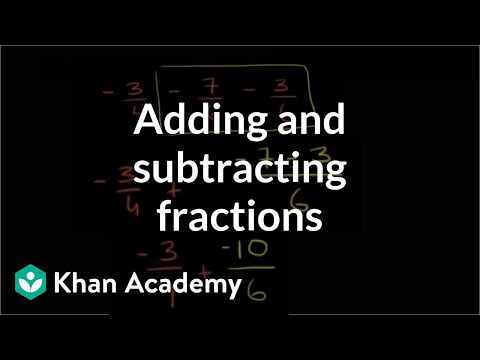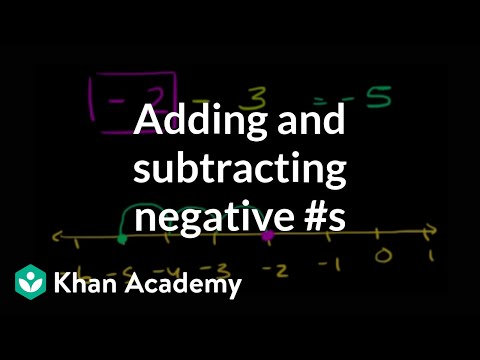Video

# Number equations & number lines (Full video)

Description: Sal writes an integer equations to describe a number line diagram. We're told to fill in the blanks to complete the equation that describes the diagram. Well we see it puts us one, two spaces to the left of zero and each of these spaces in this diagram are one. So two spaces to the left of zero is going to be negative two.

### Other videos you might be interested in### Adding & subtracting fractions (Full video)